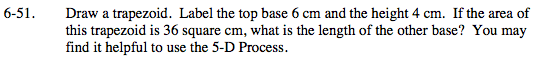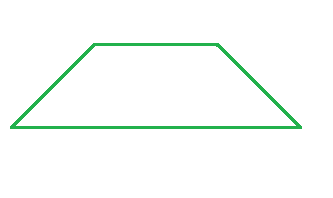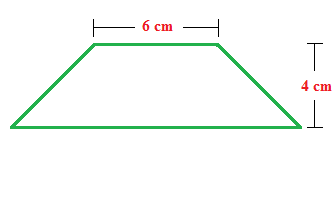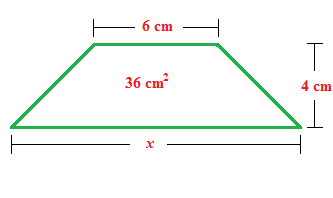### Home > MC2 > Chapter 6 > Lesson 6.1.5 > Problem6-51

6-51.Recall the area equation of a trapezoid.

$\text{Area}=\frac{1}{2}(b_1+b_2)(h)$

Plug in your known terms into the equation.

$36= \frac{1}{2} (6+x)(4)$

12 cm